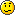## Aircraft file type for the cdu

Hi

i have some query s about the aircraft file type that we use for the CDU.

I want to write the correct values for the aircraft model that i use.

I need some help in the follow.

The parameters in the aircraft file are:

NETWeight=
Fuelmax=

the values of the airplane that i use say the follow:
All the follow values are in pounds

Empty weight : 314,000 pounds
Fuel : 267,836.81 pounds
Cross weight : 642,646 pounds
Max.gross weight : 656,000 pounds
Max allowable fuel : 269,339.99 pounds

--------------
1.The aircraft file type need pounds or kg or something else.?
2.the follow equivalence is correctNetWeight is the Empty weight ------------>314,000 pounds
Fuelmax is the Max allowable fuel ---------->269,339 pounds

-------------------------------------

And in the follow text what means the values that i have add the arrows?
I don`t understand what they mean.
These CSPD1=1 - CHDG2=1 - CHDG5=1 - CALT6=1 are necessary?

ASPD1=ARM
CSPD1=1 <---------------------------------
ASPD2=THR REF
ASPD3=SPD
ASPD4=IDLE
ASPD5=HOLD
ASPD6=GA
ASPD7=FMC SPD
AHDG1=HDG SEL
AHDG2=VOR/LOC
CHDG2=1 <----------------------------------
AHDG3=VOR/LOC
AHDG4=VOR/LOC
AHDG5=VOR/LOC
CHDG5=1 <----------------------------------
AHDG8=LNAV
AALT1=G/S
AALT2=ALT HOLD
AALT3=MCP SPD
AALT4=V/S
AALT5=ALT CAP
AALT6=G/S
CALT6=1 <----------------------------------
AALT7=TO/GA
AALT8=TO/GA
AALT9=VNAV SPD
ACMD1=A/P
ACMD2=FLT DIR
ACMD3=LAND 2
ACMD4=LAND 3
ACMD5=NO AUTOLAND
SALT1=TEST

Best Regards
Lambis
Greece